# Rectilinear Motion Formulae for NEET

When we require only one co-ordinate axis along with time to describe the motion of a particle it is said to be in linear motion or rectilinear motion. Some examples of linear motion are a parade of soldiers, a train moving along a straight line and many more.

Average Velocity

Vav = Total displacement/Total time taken = (rf – ri)/∆t

Instantaneous Velocity (at an instant)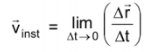Average acceleration (at an interval)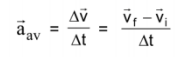Instantaneous Acceleration (at an instant)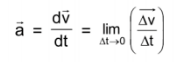v is the velocity and t is the time

Equation of motion for constant acceleration

1. v=u + at
2. s= ut + ½ at2
3. v2 = u2 + 2as
4. s = {(u+v)/2}t

For a freely falling bodies (u=0)

1. v =-gt
2. s= -½ gt2
3. v2 = – 2gs
4. sn = -g/2(2n-1)

Here,

v = final velocity

u = initial velocity

a = acceleration

t = time taken

s = displacement

Graph in Uniformly accelerated Motion along a straight line (a≠0)

1. x is a quadratic polynomial in terms of t. Hence x-t graph is a parabola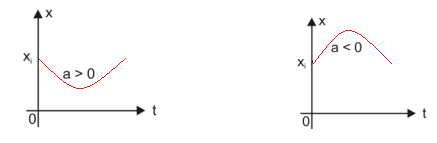1. v is a linear polynomial in terms of t. Hence v-t graph is a straight line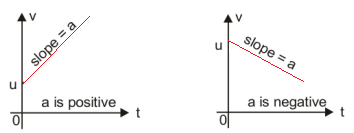1. a-t graph is a horizontal line because a is a constant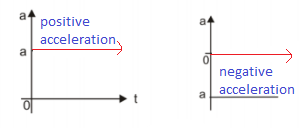Check these too: